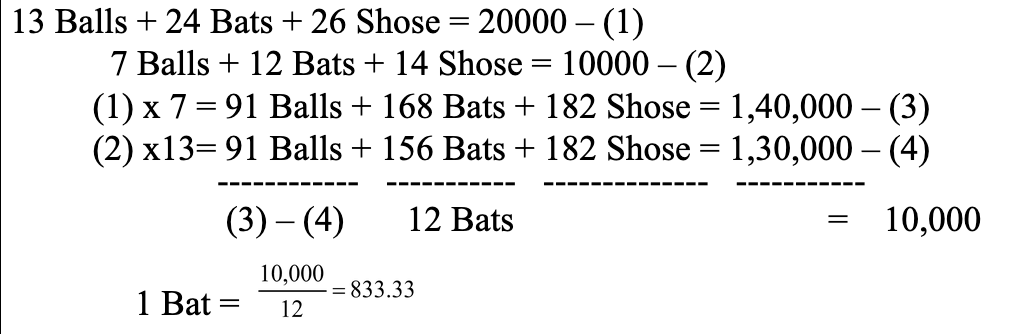## IPMAT Quants QuestionsJobs By Batch
Jobs By Location
Jobs By Degree
Jobs By Branch
IT Jobs
Internships
Govt. Jobs• ##### Original Questions of IT Companies| All Topics### Quiz Begins Here

Q #1
:

#### Quants Question

Ahmed promised to pay Balaji Rs. 8400 and certain amount after 24 months called as �pagadi� for working for him for 2 years. When Balaji quit 8 months before the agreed time, Ahmed paid him Rs. 4500 plus the entire pagadi as compensation. How much was the pagadi (in Rs.). If Balaji received all monies due to him in the appropriate proportion?
+

Let the pagadi amount be ‘p’

2/3 x (8400 + p) = 4500 + p

16800 + 2p = 13500 + 3p

p = 16800 – 13500

p = 3300

Q #2
:

#### Quants Question

Three friends, returning from a movie, stopped to eat at a restaurant. After dinner, they paid their bill and noticed of mints at the front counter. Sita took 1/3 of the mints, but returned four because she had a momentary pang of guilt. Fatima then took 1/4 of what was left but returned three for similar reasons. Eswari then took half of the remainder but threw two back into the bowl. The bowl had only 17 mints left when the raid was over. How many mints were originally in the bowl?
+

The number of mints must be divisible by 3.

Q #3
:

#### Quants Question

Rs. 800 becomes Rs. 956 in 3 years at a certain rate of simple interest. If the rate of interest is increased by 4%, what amount will Rs. 800 become in 3 years.
+

S.I. = 956 - 800 = Rs 156 R=156?100800?3R=612% New Rate = 612+4=212% New S.I. = 800�212�3100=252 Now amount will be 800 + 252 = 1052

Q #4
:

#### Quants Question

x and y are real numbers satisfying the conditions 2 < x < 3 and � 8 < y < �7. Which of the following expressions will have the least value?
+

Substitute possible figures in the given choices. In choice (a) x can be 2.9 and y can be � 6.9. In choice (c) this becomes 5(xy) which is less than x2y.

Q #5
:

#### Quants Question